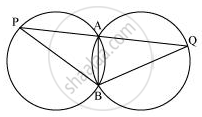# Two congruent circles intersect each other at points A and B. Through A any line segment PAQ is drawn so that P, Q lie on the two circles. Prove that BP = BQ. - Mathematics

Two congruent circles intersect each other at points A and B. Through A any line segment PAQ is drawn so that P, Q lie on the two circles. Prove that BP = BQ.

#### SolutionAB is the common chord in both the congruent circles.

∴ ∠APB = ∠AQB

In ΔBPQ,

∠APB = ∠AQB

∴ BQ = BP (Angles opposite to equal sides of a triangle)

Is there an error in this question or solution?
Chapter 10: Circles - Exercise 10.6 [Page 186]

#### APPEARS IN

NCERT Class 9 Maths
Chapter 10 Circles
Exercise 10.6 | Q 9 | Page 186

Share